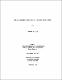## Gas breakthrough in compacted Avonlea bentoniteSeveral processes may generate gas in the proposed Canadian nuclear fuel waste disposal vault. Since a bentonite-based buffer is one of the proposed vault sealing materials, an understanding of gas migration through clay is important. Using specially-designed systems and five-minute pressure increments, the pressures required to pass gas through compacted Avonlea bentonite specimens (the gas-breakthrough pressures) were studied. Tests were conducted on saturated and unsaturated plugs with dry densities $(\rho\rm\sb{c})$ between 0.6 and 1.5 Mg/m$\sp3$. The breakthrough pressure $\rm(p\sb{b})$ of unsaturated specimens was measured with a low-pressure apparatus; $\rm p\sb{b}$ increased with increasing degree of saturation $\rm(S\sb{r}),$ provided that $\rm S\sb{r}$ was greater than about 80%. In specimens with a high degree of saturation, only inconsistent breakthrough was obtained at $\rm\rho\sb{c} > 0.60\ Mg/m\sp3$ before the 50 MPa limit of the equipment was reached. The agreement between results from saturated clay and predictions made using models based on a capillary-pressure theory of gas breakthrough is poor. Tests were also conducted on saturated specimens at constant pressure between $\rm\rho\sb{c} = 0.8$ and 1.4 Mg/m$\sp3,$ and the time to breakthrough $\rm(t\sb{b})$ was measured. Breakthrough occurred in all of these tests, but often after a much longer time than the duration of the increasing-pressure tests. This shows that a model based on capillarity alone is insufficient to describe gas breakthrough. At 1.00 Mg/m$\sp3$ and $\rm p\sb{c} = 0.3$ to 2.8 MPa, an inverse linear relationship exists between $\rm t\sb{b}$ and $\rm p\sb{c}.$ Models of the breakthrough process that assume a hydraulic-conductivity mechanism also suggest that $\rm t\sb{b}$ and $\rm p\sb{c}$ should be inversely related. Furthermore, excellent agreement was obtained between one of the models (the Kozeny-Carman time model) and the experimental data. (Abstract shortened by UMI.)